## Hierarchical clustering of samples

A hierarchical clustering of samples is a tree representation of their relative similarity.

The tree structure is generated by

1. letting each sample be a cluster
2. calculating pairwise distances between all clusters
3. joining the two closest clusters into one new cluster
4. iterating 2-3 until there is only one cluster left (which will contain all samples).
The tree is drawn so that the distances between clusters are reflected by the lengths of the branches in the tree. Thus, features with expression profiles that closely resemble each other have short distances between them, those that are more different, are placed further apart.

(See [Eisen et al., 1998] for a classical example of application of a hierarchical clustering algorithm in microarray analysis. The example is on features rather than samples).

To start the clustering:

Toolbox | Transcriptomics Analysis ()| Quality Control | Hierarchical Clustering of Samples (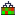)

Select a number of samples ( () or ()) or an experiment () and click Next.

This will display a dialog as shown in figure 28.86. The hierarchical clustering algorithm requires that you specify a distance measure and a cluster linkage. The similarity measure is used to specify how distances between two samples should be calculated. The cluster distance metric specifies how you want the distance between two clusters, each consisting of a number of samples, to be calculated.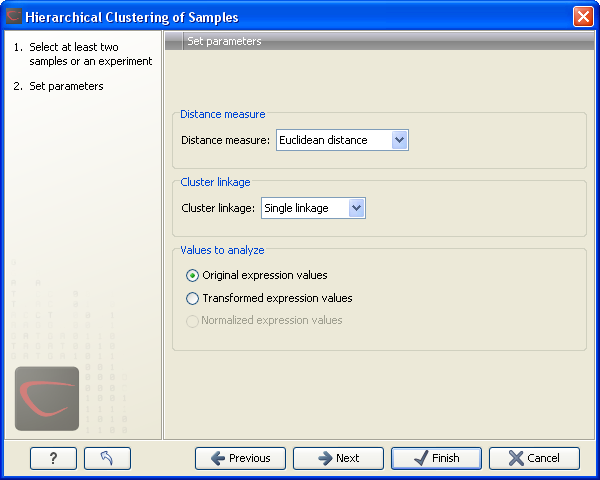Figure 28.86: Parameters for hierarchical clustering of samples.

At the top, you can choose three kinds of Distance measures:

• Euclidean distance. The ordinary distance between two points - the length of the segment connecting them. If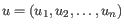and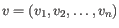, then the Euclidean distance between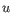and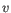is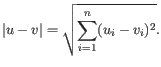• 1 - Pearson correlation. The Pearson correlation coefficient between two elements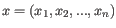and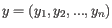is defined as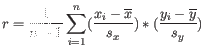where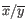is the average of values in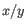and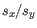is the sample standard deviation of these values. It takes a value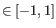. Highly correlated elements have a high absolute value of the Pearson correlation, and elements whose values are un-informative about each other have Pearson correlation 0. Using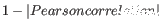as distance measure means that elements that are highly correlated will have a short distance between them, and elements that have low correlation will be more distant from each other.
• Manhattan distance. The Manhattan distance between two points is the distance measured along axes at right angles. Ifand, then the Manhattan distance betweenandis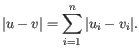Next, you can select the cluster linkage to be used:

• Single linkage. The distance between two clusters is computed as the distance between the two closest elements in the two clusters.
• Average linkage. The distance between two clusters is computed as the average distance between objects from the first cluster and objects from the second cluster. The averaging is performed over all pairs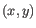, where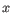is an object from the first cluster and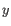is an object from the second cluster.
• Complete linkage. The distance between two clusters is computed as the maximal object-to-object distance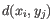, where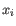comes from the first cluster, and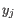comes from the second cluster. In other words, the distance between two clusters is computed as the distance between the two farthest objects in the two clusters.

At the bottom, you can select which values to cluster (see Selecting transformed and normalized values for analysis).

Click Next if you wish to adjust how to handle the results. If not, click Finish.

Note: the workflow execution of this tool does not end up modifying the input experiment. Instead, a standalone heat map is created.

Subsections